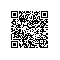# Swift静态方法

struct Account {

var owner : String = "Tony"                 //账户名	①
static var interestRate : Double = 0.668    //利率	②

static func interestBy(amount : Double) -> Double {	③

return interestRate * amount
}

func messageWith (amount : Double) -> String {	④

var interest = Account.interestBy(amount)
return "\(self.owner) 的利息是\(interest)"

}
}

//调用静态方法
println(Account.interestBy(10_000.00))	⑤

var myAccount = Account()	⑥
//调用实例方法
println(myAccount.messageWith (10_000.00))	⑦

enum Account {

case 中国银行
case 中国工商银行
case 中国建设银行
case 中国农业银行

static var interestRate : Double = 0.668 //利率	①

static func interestBy(amount : Double) -> Double {	②
return interestRate * amount
}
}

//调用静态方法
println(Account.interestBy(10_000.00 )) 	③

class Account {

var owner : String = "Tony"                 //账户名

class func interestBy(amount : Double) -> Double {	①
return 0.8886 * amount
}
}

//调用静态方法
println(Account.interestBy(10_000.00 ))	②使用钉钉扫一扫加入圈子
+ 订阅